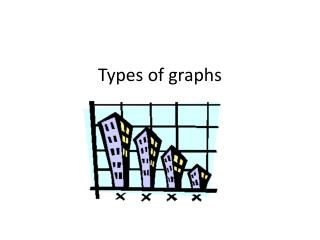DownloadDownload PresentationTypes of graphs

# Types of graphs

Download Presentation## Types of graphs

- - - - - - - - - - - - - - - - - - - - - - - - - - - E N D - - - - - - - - - - - - - - - - - - - - - - - - - - -
##### Presentation Transcript

1. Types of graphs

2. Graphs are pictures that help us understand amounts. These amounts are called data. There are many kinds of graphs, each having special parts.

3. circle • A circle graph is shaped like a circle. It is divided into fractions that look like pieces of pie, so sometimes a circle graph is called a pie graph. Many times the fractional parts are different colors and a key explains the colors.

4. Bar graph • A bar graph uses bars to show data. The bars can be vertical (up and down), or horizontal (across). The data can be in words or numbers.

5. Picture graph • A picture graph uses pictures or symbols to show data. One picture often stands for more than one vote so a key is necessary to understand the symbols.

6. historgram • A histogram is a special kind of bar graph. The data must be shown as numbers in order.

7. Line graph • A line graph shows points plotted on a graph. The points are then connected to form a line.

8. Frequency table • A frequency table shows the number of times a list of items occur, with the use of tally marks.

9. Stem and leaf plot • The "stem" is the left-hand column which contains the tens digits. The "leaves" are the lists in the right-hand column, showing all the ones digits for each of the tens, twenties, thirties, and forties. As you can see, the original values can still be determined; you can tell, from that bottom leaf, that the three values in the forties were 40, 40, and 41.

10. Scatter plot • A scatter plot displays two related sets of data that are measured or counted

11. DONE! • The End!